# Coordinates in ggplot2 in R

The coordinate system of a plot refers to the space on which the data is plotted. The coordinate system of a plot, together with the x and y position scale, determines the location of geoms.

Below are the available coordinate options:

• coord_cartesian: This is the default coordinate system (x horizontal from left to right, y vertical from bottom to top)
• coord_flip: Flipped cartesian coordinate system (x vertical from bottom to top, y horizontal from left to right)
• coord_trans: Used to to transform the coordinate system. We, i.e., substitute traditional axes with logarithmic axes and then present the values and statistics
• coord_equal: Ensures the units are equally scaled on the x-axis and on the y-axis
• coord_polar: Polar coordinate system; the x (or y) scale is mapped to the angle (theta)
• coord_map: Various map projections

Most popular graphs such as line and bar charts are drawn using Cartesian coordinates.

### coord_cartesian

When we plot a chart, Cartesian Coordinates is the default coordinate system. For example, the following chart plots Duration of Credit on x-axis and Credit Amount on y-axis. The points are colors by the factor Loan.Quality.

g <- ggplot(df,aes(x=Duration.of.Credit..in.months.,y=Credit.amount,color=Loan.Quality))
g+geom_point()+geom_smooth()+coord_cartesian()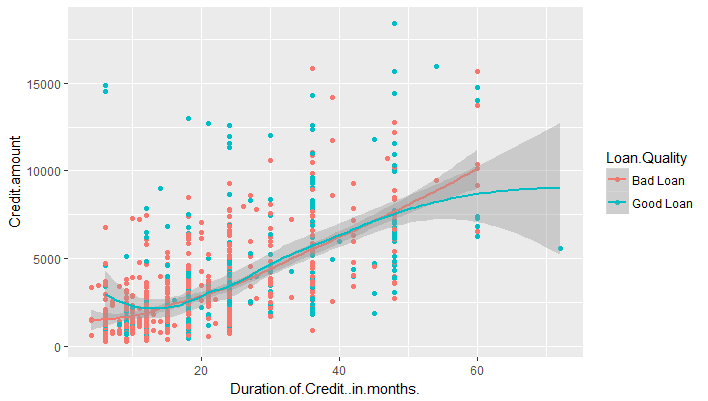### coord_flip

By specifying coord_flip, it will still use the cartesian system, but flip the two axis.

g <- ggplot(df,aes(x=Duration.of.Credit..in.months.,y=Credit.amount,color=Loan.Quality))
g+geom_point()+geom_smooth()+coord_flip()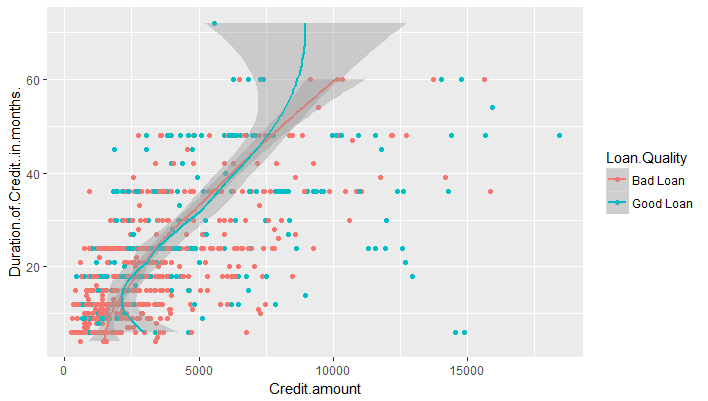### Zooming In

We can use the coordinates layer to zoom in to a plot by setting limits. The various options are below:

coord_cartesian(xlim = NULL, ylim = NULL, expand = TRUE)

• xlim, ylim: Limits for the x and y axes.
• expand: If TRUE, the default, adds a small expansion factor to the limits to ensure that data and axes don't overlap. If FALSE, limits are taken exactly from the data or xlim/ylim

Let's say we want to zoom in our plot to show the points where the Duration of credit is between 40 and 60. This can be done as follows:

g <- ggplot(df,aes(x=Duration.of.Credit..in.months.,y=Credit.amount,color=Loan.Quality))
g+geom_point()+geom_smooth()+coord_cartesian(c(40,60))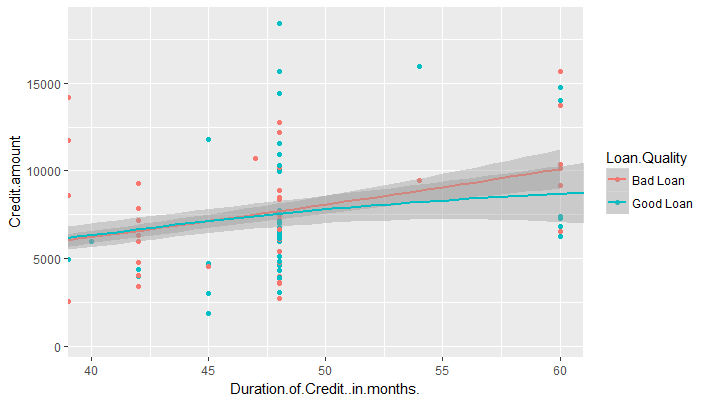### Aspect Ratio

We can set the aspect ratio of a plot with coord_fixed() or coord_equal(). Both use aspect = 1 (1:1) as a default. For example, consider the following plot which plots Duration of Credit on x-axis and Age on y-axis.

g <- ggplot(df,aes(x=Duration.of.Credit..in.months.,y=Age.in.years,color=Loan.Quality))
g+geom_point()+geom_smooth()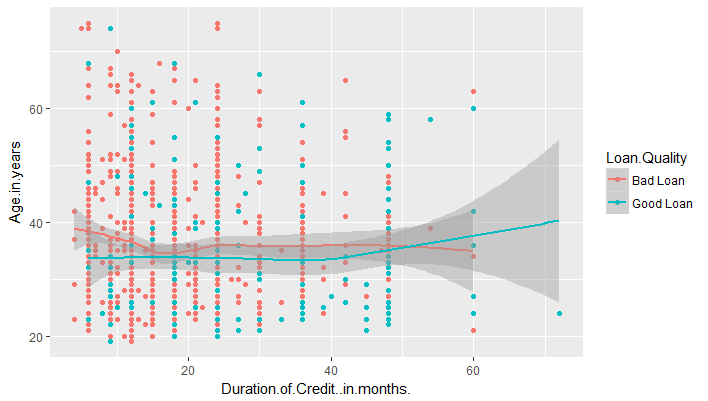We can add the coordinate coord_equal() to make the aspect ratio 1:1.

g <- ggplot(df,aes(x=Duration.of.Credit..in.months.,y=Age.in.years,color=Loan.Quality))
g+geom_point()+geom_smooth()+coord_equal()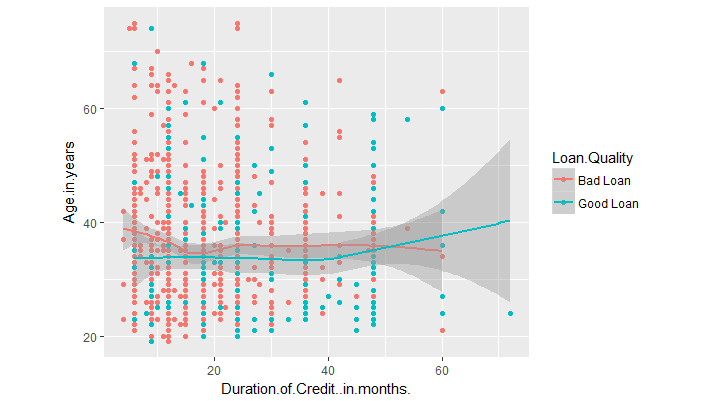Get our Data Science for Finance Bundle for just $29$51.